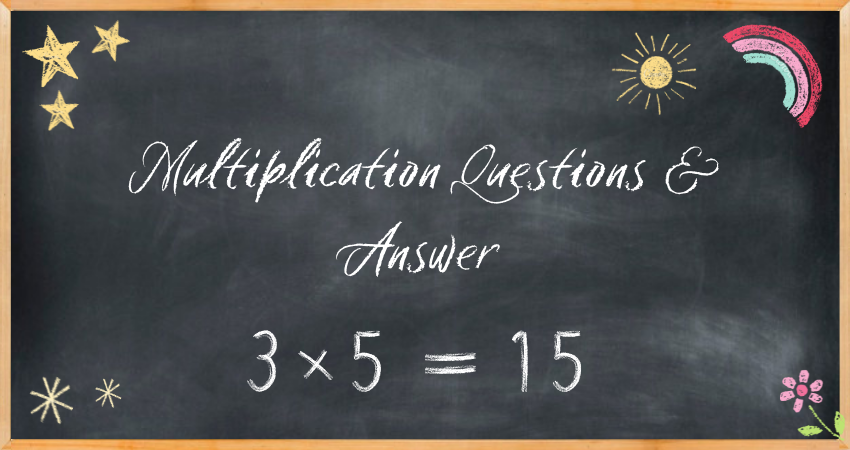Online Multiplication Questions with Answers | Best Kids Learning Platform - Easyshiksha

# Maths Multiplication Questions With Answers• 2x2 = ?
• 3x2 = ?
• 3x3 = ?
• 2x4 = ?
• 2x5 = ?
• 11x7 = ?
• 10x2 = ?
• 13x4 = ?
• 15x3 = ?
• 17x5 = ?
• 17x12 = ?
• 15x18 = ?
• 22x27 = ?
• 25x32 = ?
• 27x19 = ?
• 124x25 = ?
• 237x34 = ?
• 385x53 = ?
• 489x16 = ?
• 358x42 = ?